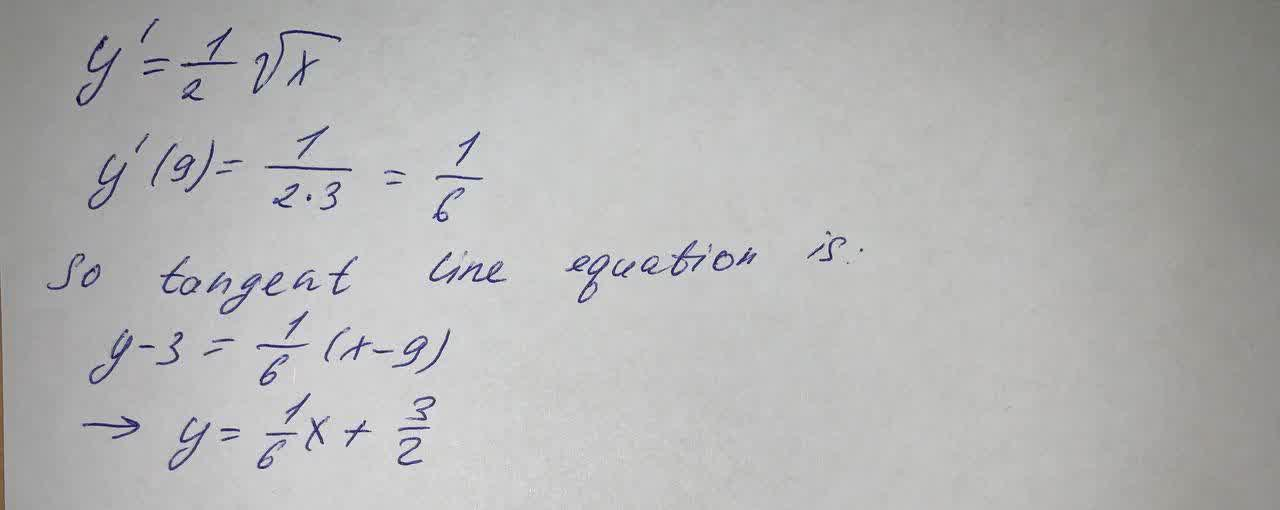Question# Find an equation of the tangent line to the curve at the given point (9, 3) y=\frac{1}{\sqrt{x}}

Differential equations
ANSWERED$$y=\frac{1}{\sqrt{x}}$$